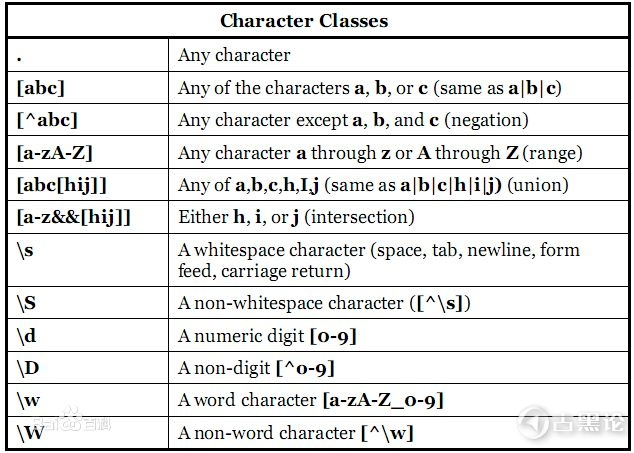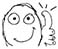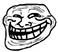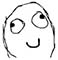# [# 其他] sorry, 会正则真的可以为所欲为1.元字符

 元字符 说明 . 匹配除换行符以外的任意字符 \w 匹配字母或数字或下划线或汉字 \s 匹配任意的空白符 \d 匹配数字 \b 匹配单词的开始或结束 ^ 匹配字符串的开始 \$ 匹配字符串的结束

`\babc`

`^abc`

`^\d\d\d\d\d\d\d\d\$`

`^1\d\d\d\d\d\d\d\d\d\d\$`

2. 重复限定符

 语法 说明 * 重复零次或更多次 + 重复一次或更多次 ? 重复零次或一次 {n} 重复n次 {n,} 重复n次或更多次 {n,m} 重复n到m次

`^\d{8}\$`

`^1\d{10}\$`

`^\d{14,18}\$`

`^ab*\$`

3. 分组

`^(ab)*`

4. 转义

`^(\(ab\))*`

5. 条件或

`^(130|131|132|155|156|185|186|145|176)\d{8}\$`

6. 区间

`^((13[0-2])|(15)|(18[5-6])|145|176)\d{8}\$`1. 零宽断言

`"<span class="read-count">阅读数：641</span>"`

``` String reg=".+(?=</span>)";
Pattern pattern = Pattern.compile(reg);
Matcher mc=    pattern.matcher(test);
while(mc.find()){
System.out.println("匹配结果：")
System.out.println(mc.group());
}

//匹配结果：

```String reg="\\d+(?=</span>)";
Pattern pattern = Pattern.compile(reg);
Matcher mc=    pattern.matcher(test);
while(mc.find()){
System.out.println(mc.group());
}```

``` //(?<=<span class="read-count">阅读数：)\d+
Pattern pattern = Pattern.compile(reg);
Matcher mc=    pattern.matcher(test);
while(mc.find()){
System.out.println(mc.group());
}

//匹配结果：
//641```

`祖国(?!的花朵)`

2. 捕获和非捕获

 序号 编号 分组 内容 0 0 (0\d{2})-(\d{8}) 020-85653333 1 1 (0\d{2}) 020 2 2 (\d{8}) 85653333

``` String test = "020-85653333";
String reg="(0\\d{2})-(\\d{8})";
Pattern pattern = Pattern.compile(reg);
Matcher mc= pattern.matcher(test);
if(mc.find()){
System.out.println("分组的个数有："+mc.groupCount());
for(int i=0;i<=mc.groupCount();i++){
System.out.println("第"+i+"个分组为："+mc.group(i));
}
}```

```分组的个数有：2

 序号 名称 分组 内容 0 0 (0\d{2})-(\d{8}) 020-85653333 1 quhao (0\d{2}) 020 2 haoma (\d{8}) 85653333

```String test = "020-85653333";
String reg="(?<quhao>0\\d{2})-(?<haoma>\\d{8})";
Pattern pattern = Pattern.compile(reg);
Matcher mc= pattern.matcher(test);
if(mc.find()){
System.out.println("分组的个数有："+mc.groupCount());
System.out.println(mc.group("quhao"));
System.out.println(mc.group("haoma"));
}```

```分组的个数有：2

`(?:\0\d{2})-(\d{8})`

 序号 编号 分组 内容 0 0 (0\d{2})-(\d{8}) 020-85653333 1 1 (\d{8}) 85653333

```String test = "020-85653333";
String reg="(?:0\\d{2})-(\\d{8})";
Pattern pattern = Pattern.compile(reg);
Matcher mc= pattern.matcher(test);
if(mc.find()){
System.out.println("分组的个数有："+mc.groupCount());
for(int i=0;i<=mc.groupCount();i++){
System.out.println("第"+i+"个分组为："+mc.group(i));
}
}```

```分组的个数有：1

3. 反向引用

1）匹配到一个字母
2）匹配第下一个字母，检查是否和上一个字母是否一样
3）如果一样，则匹配成功，否则失败

```String test = "aabbbbgbddesddfiid";
Pattern pattern = Pattern.compile("(\\w)\\1");
Matcher mc= pattern.matcher(test);
while(mc.find()){
System.out.println(mc.group());

}```

```aa
bb
bb
dd
dd
ii```

```String test = "abcbbabcbcgbddesddfiid";
String reg="(a)(b)c";
System.out.println(test.replaceAll(reg, "\$1"));;```

`abbabcgbddesddfiid`

4. 贪婪和非贪婪

1.贪婪

`\d{3,6}`

```String reg="\\d{3,6}";
String test="61762828 176 2991 871";
System.out.println("文本："+test);
System.out.println("贪婪模式："+reg);
Pattern p1 =Pattern.compile(reg);
Matcher m1 = p1.matcher(test);
while(m1.find()){
System.out.println("匹配结果："+m1.group(0));
}```

```文本：61762828 176 2991 44 871

```String reg="(\\d{1,2})(\\d{3,4})";
String test="61762828 176 2991 87321";
System.out.println("文本："+test);
System.out.println("贪婪模式："+reg);
Pattern p1 =Pattern.compile(reg);
Matcher m1 = p1.matcher(test);
while(m1.find()){
System.out.println("匹配结果："+m1.group(0));
}
```

```文本：61762828 176 2991 87321

“617628” 是前面的\d{1,2}匹配出了61，后面的匹配出了7628
"2991" 是前面的\d{1,2}匹配出了29 ，后面的匹配出了91
"87321"是前面的\d{1,2}匹配出了87，后面的匹配出了321

2. 懒惰（非贪婪）

 代码 说明 *? 重复任意次，但尽可能少重复 +? 重复1次或更多次，但尽可能少重复 ?? 重复0次或1次，但尽可能少重复 {n,m}? 重复n到m次，但尽可能少重复 {n,}? 重复n次以上，但尽可能少重复

```String reg="(\\d{1,2}?)(\\d{3,4})";
String test="61762828 176 2991 87321";
System.out.println("文本："+test);
System.out.println("贪婪模式："+reg);
Pattern p1 =Pattern.compile(reg);
Matcher m1 = p1.matcher(test);
while(m1.find()){
System.out.println("匹配结果："+m1.group(0));
}```

```文本：61762828 176 2991 87321

“61762” 是左边的懒惰匹配出6，右边的贪婪匹配出1762
"2991"  是左边的懒惰匹配出2，右边的贪婪匹配出991
"87321" 左边的懒惰匹配出8，右边的贪婪匹配出7321

5. 反义

 元字符 解释 \W 匹配任意不是字母，数字，下划线，汉字的字符 \S 匹配任意不是空白符的字符 \D 匹配任意非数字的字符 \B 匹配不是单词开头或结束的位置 [^x] 匹配除了x以外的任意字符 [^aeiou] 匹配除了aeiou这几个字母以外的任意字符

### 评分小执念 + 10 + 10 简单来说，这件事一点不简单。.纯粹路过，没任何兴趣，仅仅是看在金币份上回复一下ps:楼主别打我，我是小白就艺术的角度而言，这篇帖子已然为经典之作，但它的意义却远远大于经典本身。正所谓：“骂而无形，讥于无影，笑骂之中显真功！”楼主真不愧为讽刺界新一代的开山长毛鼻祖！hhjj543212012「龙战于野」 2019-2-25 08:10 |显示全部楼层

 顶顶顶有用，收藏一个您需要登录后才可以回帖 登录 | 注册账号   本版积分规则 回帖后跳转到最后一页

GMT+8, 2020-7-16 00:59 , Processed in 0.037970 second(s), 22 queries , Redis On.